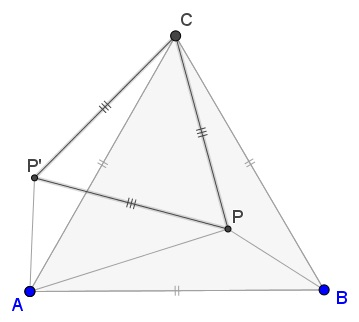# Luca Moroni's Problem In Equilateral Triangle

### Problem### Solution 1

Let $R_{C}$ be the clockwise rotation around $C$ through $60^{\circ}.$ Then $R_{C}(B)=A$ and let $P'=R_C(P).$Note that in $\Delta APP',$ $PP'=PC$ and $AP'=PB$ so that $APP'$ is the sought triangle.

Denote $\angle APC=\alpha$ and $\angle APB=\beta.$ Observe that $R_{C}(\Delta BPC)=\Delta AP'C,$ so that $\angle AP'C=360^{\circ}-\alpha-\beta,$ implying $\angle AP'P=300^{\circ}-\alpha-\beta.$ Further, $\angle APP'=\alpha-60^{\circ}$ so that

\begin{align}\angle PAP'&=180^{\circ}-(300^{\circ}-\alpha-\beta)-(\alpha-60^{\circ})\\ &=\beta-60^{\circ}. \end{align}

In particular case where $\alpha=100^{\circ}$ and $\beta=120^{\circ},$

$\angle PAP'=120^{\circ}-60^{\circ}=60^{\circ},\\ \angle AP'P=300^{\circ}-\alpha-\beta=80^{\circ},\\ \angle APP'=\alpha-60^{\circ}=40^{\circ}.$

### Solution 2

WLOG, let $AB=1$. Let $PA=a,~PB=b,~PC=c$ and $\angle PAB=\phi$. Thus, $\angle PAC=\angle PBA=60^{\circ}-\phi$, $\angle PBC=\phi$.

From sine rule applications,

\begin{align} & a\sin 120^{\circ}=\sin (60^{\circ}-\phi) ~(\Delta PAB) \\ &b\sin 120^{\circ}=\sin \phi~(\Delta PAB) \\ &c\sin 100^{\circ}=\sin(60^{\circ}-\phi)~(\Delta PAC) \\ &c\sin 140^{\circ}=\sin \phi~(\Delta PBC) \end{align}

Consolidating the four equations into two by eliminating the common unknown sines,

\begin{align} &a\sin 120^{\circ}=c\sin 100^{\circ} \\ &b\sin 120^{\circ}=c\sin 140^{\circ}. \end{align}

Thus,

$\displaystyle \frac{a}{\sin 100^{\circ}}=\frac{b}{\sin 140^{\circ}}=\frac{c}{\sin 120^{\circ}}.$

Using the supplementary angles,

$\displaystyle \frac{a}{\sin 80^{\circ}}=\frac{b}{\sin 40^{\circ}}=\frac{c}{\sin 60^{\circ}}.$

Note, the three angles add up to $180^{\circ}$. Thus, what we have is the sine rule for a triangle with angles $40^{\circ}, 60^{\circ}, 80^{\circ}$ and opposite sides $b,c,a$, respectively.

### Acknowledgment

This problem was posted on twitter by Luca Moroni. Solution 2 is by Amit Itagi.